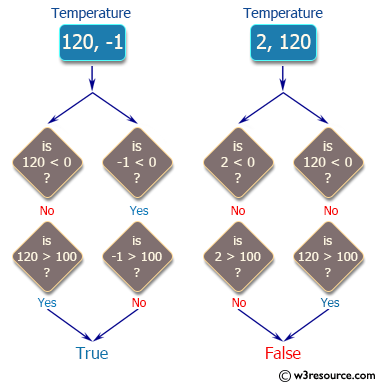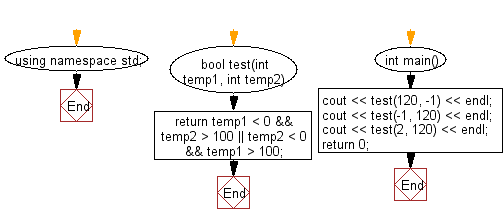﻿ C++ Exercises: Check if one given temperatures is less than 0 and the other is greater than 100 - w3resource# C++ Exercises: Check if one given temperatures is less than 0 and the other is greater than 100

## C++ Basic Algorithm: Exercise-13 with Solution

Write a C++ program to check if one given temperatures is less than 0 and the other is greater than 100.

Sample Solution:

C++ Code :

``````#include <iostream>
using namespace std;

bool test(int temp1, int temp2)
{
return temp1 < 0 && temp2 > 100 || temp2 < 0 && temp1 > 100;
}

int main()
{
cout << test(120, -1) << endl;
cout << test(-1, 120) << endl;
cout << test(2, 120) << endl;
return 0;
}
``````

Sample Output:

```1
1
0
```

Pictorial Presentation:Flowchart:C++ Code Editor:

Contribute your code and comments through Disqus.

What is the difficulty level of this exercise?

﻿